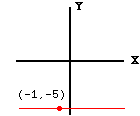Date: Wed, 23 Sep 1998 13:32:23 -0600 (CST)
To: QandQ@MathCentral.uregina.ca
Subject: linear equations

Name: tess

Who is asking: Student
Level: Secondary

Question:
Find the equations of a line through (-1,-5) and parallel to the X-axis.

Hi Tess,

 The properties of the required line are that it is parallel to the X-axis and that it passes through (-1,-5). From the diagram it is clear that every point on the line is the same distance from the X-axis. That is evert point on the line has the same Y co-ordinate. Since the Y co-ordinate of the given point is -5 a point is on th line if and only its Y co-ordinate is -5. Hence the equation of the line is y  = -5. Cheers, HarleyGo to Math Central

To return to the previous page use your browser's back button.